Smartick is a fun way to learn math!Feb04

# Examples of Second Grade Word Problems with Solutions

In today’s post, we are going to see a selection of Second Grade word problems that usually appear at the end of a Smartick session for children with a second-grade level. You will also get a chance to see the solution for each problem.

Index

## How To Correctly Solve Any Problem

• Read the word problem carefully! You should understand what is being asked very clearly.
• Write down the data.
• Complete the operations and always write down the results for each.
• Write the solution very clearly and respond to what the problem asks.

Let’s practice with a selection of Second Grade word problems from Smartick.

### Word Problem 1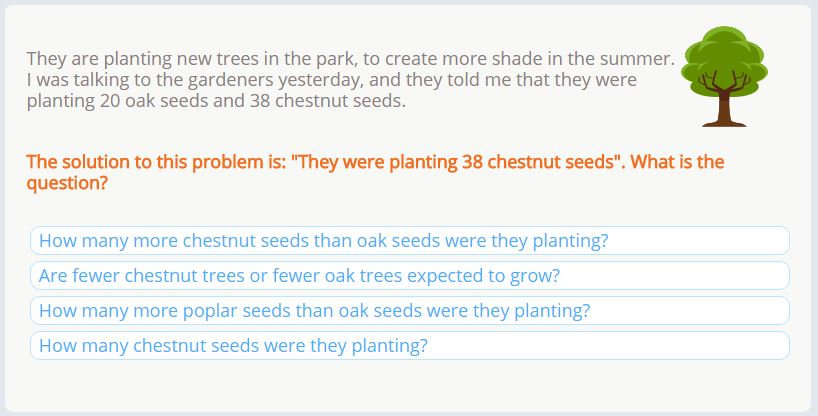#### Solution 1

For this word problem, Smartick has given us the solution and it is up to us to select the correct question.

The answer is: ”They were planting 38 chestnut seeds.” Let’s take a look at each option to see which would work with this answer, we’ll use the process of elimination.

• How many more chestnut seeds than oak seeds were they planting? This is asking us about the different types of seeds, but the solution is only asking about chestnuts so this question is not what we are looking for.
• Are fewer chestnut trees or fewer oak trees expected to grow? This answer isn’t the one we are looking for because the answer only gives us information about chestnut seeds.
• How many more poplar seeds than oak seeds were they planting? Nothing was mentioned in the word problem or the answer about poplar trees.
• How many chestnut seeds were they planting? Here were are being asked about the number of chestnut seeds that were planted and the solution tells us the amount that was planted. This is the question that we are looking for.

### Word Problem 2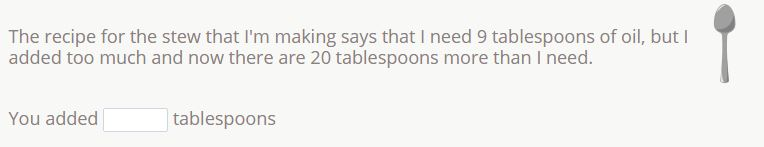#### Solution 2

This word problem is asking how many tablespoons of oil have been added to a stew, and we know that there were 20 tablespoons more added than the 9 tablespoons that the recipe called for. So we need to know how many tablespoons in total were added to the stew.

9 + 20 = 29

29 tablespoons of oil were added to the stew.

### Word Problem 3#### Solution 3

Here, we are being asked to calculate the total number of clocks. To help us solve it, we can create a drawing using the information from the word problem. It tells us that there are 2 clocks on each shelf, and there are 6 shelves.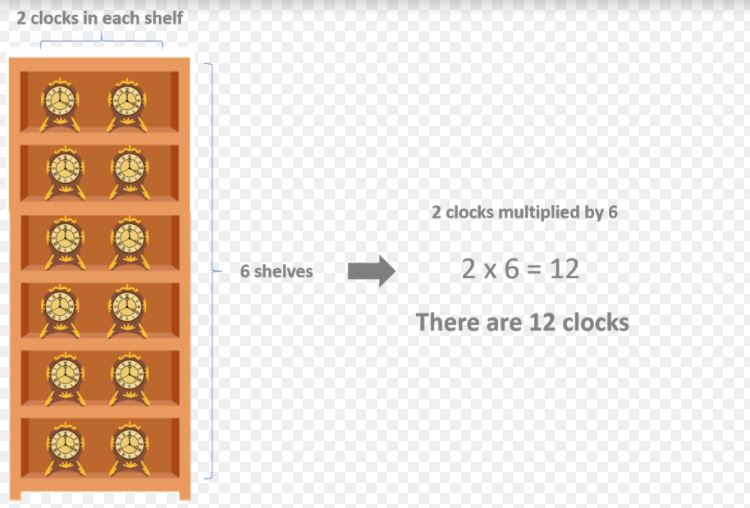### Word Problem 4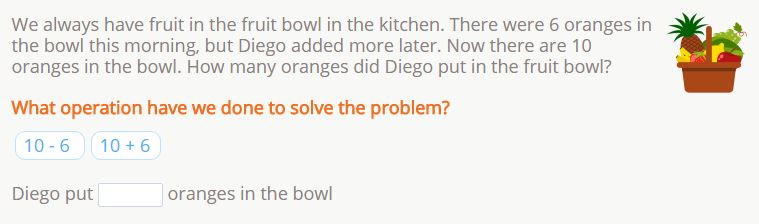#### Solution 4

First, we are asked which operation we need to use to solve the problem and are provided with the options of addition and subtraction. Let’s read the word problem carefully to see which would work best…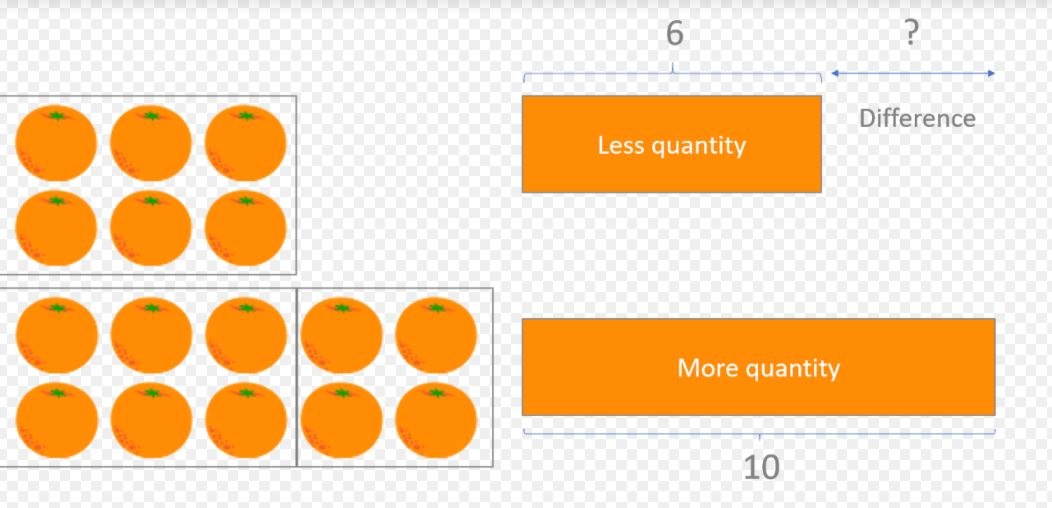There were 6 oranges before Diego added more, and now there are 10. Therefore, the difference between the amount in the bowl now, and the amount there was before, is the amount that Diego added. If we add the numbers, adding the larger quantity to the lesser quantity, it does not give us the number of oranges Diego added to the bowl. However, if we subtract the smaller quantity from the larger quantity, we should find the difference.

The answer to the first question would be: 10 – 6.

And the solution to the word problem: 10 – 6 = 4.